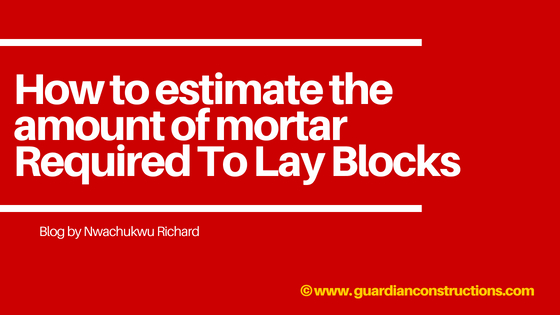# How To Estimate The Amount Of Cement Mortar Required To Lay Blocks

As a builder, experienced site engineer, i can tell you on this page that one of most difficult moment of a building contractor or a builder is that moment when the client asks “How many Blocks will i need to build my 2 bedroom flat?” or How many bags of cement will i need to mold blocks or How many bags of cement will i need to lay the block. A this point, most contractors become stunned, to save the day, they quickly say “I’ll get back to you”. With this post today, you’ll be learning How to estimate the amount of mortar Required To Lay Blocks.
This means that by the time you’re done reading this post, you will be able to answer questions like: How many bags of cement do i need to lay the blocks? How many trips of sand do i need for the block workJust sit back and learn with me.

## How Many Blocks are there in One square meter?

When i wrote this article on how many block are there in 1 square meter, i explained that there are about 10 number of blocks in every 1 square meter.
Want More proof?
Ok, walk with me dear.

## What is the area of one 9 inch block in Nigeria?

In Nigeria, the area of a 9 inch block = 450mm x 225mm. The product of this gives us 0.101m2 isn’t it?

Now to get the number of blocks to cover 1 square meter, we simply divide 1m2 by 0.101.
This gives us 9.99 isn’t it?
Can we just assume its 10 blocks since we cannot have 9.9 blocks?
Before we proceed, lets quickly take a good look at the dimensions of a 9 inch block with voids (9 is very popular, known because it is usually used for building in Nigeria)
Having seen the above image comprising of the various sections of a 9 inch hollow block, lets calculate the area of the block that will receive mortar.

How to Calculate the amount of mortar required by a 9 inch block
Ab = Area of the whole block – area of Voids
Ab = (0.45 x 0.225) – 2(0.15 x 0.125) = 0.0637 m2

## What is the required thickness of mortar?

The required thickness of mortar is usually 25mm
From the above, we can say that for every 9 inch block, there is need for 0.0637 x 25 and this gives us a 1.6m3 of mortar.
We will get back to this later on.
Now, let us assume that the mortar will be 25 mm thick
The typical arrangement of blocks in a one square meter wall is given below;
Having seen the arrangement of blocks in a square meter, the number of blocks, lets now calculate the volume of mortar for 1 square meter of block
From the vertical section of a 1 square meter wall, the volume of mortar will be:

Vertical Mortar Requirements = 8 x (0.025 x 0.225 x 0.275) = 0.0123 m3
Horizontal mortar Requirements = 10 x (0.0637 x 0.025) = 0.0159 m3

Now, Adding Vertical and Horizontal Mortar requirements, we get a total of  = 0.0282 m3

To go further, let us assume that we have 300 m2 of wall, and we want to estimate the quantity of cement and sand for mortar needed to lay the blocks.

What Volume of Mortar is required to Lay Blocks on a 300m2 wall?

The volume of mortar required = 0.03 x 300 = 9 m3

## Materials For Cement Mortar

For Cement
Quantity of cement required = 1/7 x 1440 kg/m3 = 205.71 kg
Making allowance for shrinkage between fresh and wet concrete = 1.54 x 205.71 = 316.79 kg
You Must Provide = 9 x 6.33 = 57 bags of cement + allowance for waste as appropriate.

For Sharp Sand
Quantity of sand required = 6/7 x 1600 kg/m3 = 1371.428 kg
Making allowance for shrinkage between fresh and wet concrete = 1.54 x 1371.428 = 2112 kg
For 9m3 Of mortar;
You Must Provide = 9 x 2112 = 19004 kg of sharp sand + allowance for waste as appropriate.
So you can order 20 tons of sharp sand and 57 bags of cement for this job.

Now i am sure you understand How to estimate the amount of mortar Required To Lay Blocks (Cement and Sand) covering one square meter as well as make estimates for cement mortar.
Note: This post was made using a 9 inch block as a case study, the volume of mortar will differ if you’re using a 6 inch hollow block.
Also note that this post was made with the Nigerian contractors in mind.

Hi, I'm Okpese! It is my job to handle the content aspect of this great organization and I'm determined to ensure you get it all right as long as you're handling a construction project in Nigeria!

1. Thanks for this eye Opening Boq

• Moderation?

• You are welcome Mr Wasiu

2. I wish every everyone could know how important ur contents are ….I am so happy to come across a blog like this.pls I need ur daily updates and notifications..

### Factors To Consider When Choosing Doors For Your Building